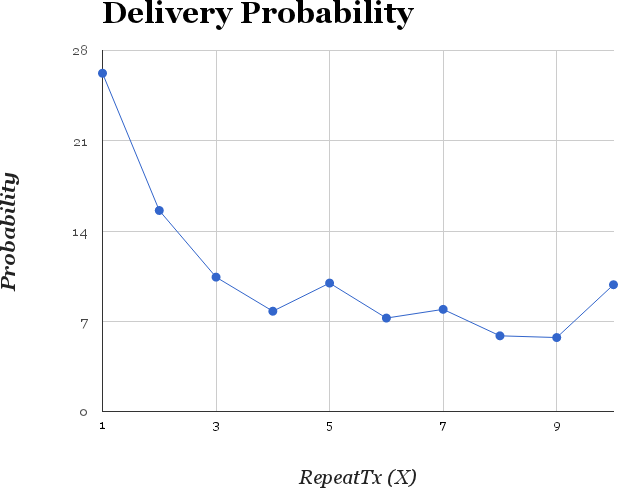Now we have designed the simulator, add a new MAC protocol to NS2, and implement the Random Resend MAC protocol, the final part will be analyze the trace file to measure the performance of our new protocol.

### Format of the Trance Entry

One line in the trace file may look like this.

```s 0.010830867 _70_ MAC  --- 27 cbr 148 [0 46000000 8 0] ------- [70:0 0:0 32 0]  0 0
|      |        |   |        |  |   |
|      |        |   |        |  |   +----- Packet Size
|      |        |   |        |  +--------- Traffic Type
|      |        |   |        +------------ Packet UID
|      |        |   +--------------------- Layer
|      |        +------------------------- Node ID
|      +---------------------------------- Time
+----------------------------------------- Event Type
```

You can find more details about the trace format here.

### Trace Filtering

In this project, we're only interested the traces that

• From MAC layer, and
• With CBR traffic type
• With Event Type in "Send"(s), "Receive"(r) and "Drop"(D)

So we first filter those not-so-interesting traces out.

```def filter(trace_file) :
with open(trace_file, 'r') as f :

print("%s raw traces found." % (len(raw_lines)))

traces = []
for line in raw_lines :
fields = line.split(' ')
if fields not in ['s', 'r', 'D'] :
continue
if not (fields == 'MAC') :
continue
if not (fields == 'cbr') :
continue

traces.append({'action': fields, 'node': fields, 'pid': int(fields)})

print("%s filtered traces found." % (len(traces)))
return traces
```

### Delivery Probability

To calculate the delivery probability, we need to know

• How many unique packets are sent out by all source nodes?
• How many unique packets received by the sink node?

These two metrics can be easily obtained as following:

```nodes = set(t['node'] for t in traces)
print("%s nodes found." % (len(nodes)))

sent = len(set(t['pid'] for t in traces))
recv = len(set(t['pid'] for t in traces if t['node'] == SINK_NODE and t['action'] == 'r'))

print("sent: %d, recv: %d, P: %.2f%%" % (sent, recv, float(recv)/sent*100))
```

Remember that we use `LossMonitor` as sink? Now is the time to cross-reference the results here with the ones from the stats file. The total received packets number should match.

The final delivery probability w.s.t the repeat count `X` is somehow like this in my case (packet size is 16 bytes).Note that somehow this is not the ideal probability distribution. Please refer to this paper for theoretical analysis and also simulation results.

QoMOR: A QoS-aware MAC protocol using Optimal Retransmission for Wireless Intra-Vehicular Sensor Networks